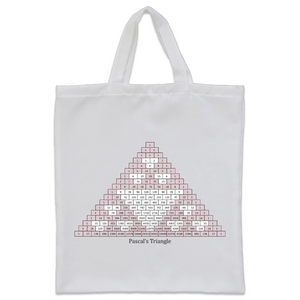# Pascal's Triangle Tote Bag

• £13.49
Unit price per
Tax included.

A tote perfect to carry teaching supplies from classroom to classroom, Pascal’s Triangle is a number pattern, named after the French mathematician Blaise Pascal. Pascal’s Triangle has many applications in mathematics and statistics, including the calculation of combinations. Pascal’s Triangle can also be used to find calulate probability, in algebra and finding triangular numbers. There are many more interesting patterns you can find in the triangle, including prime numbers, Catalan numbers, and the Fibonacci sequence.